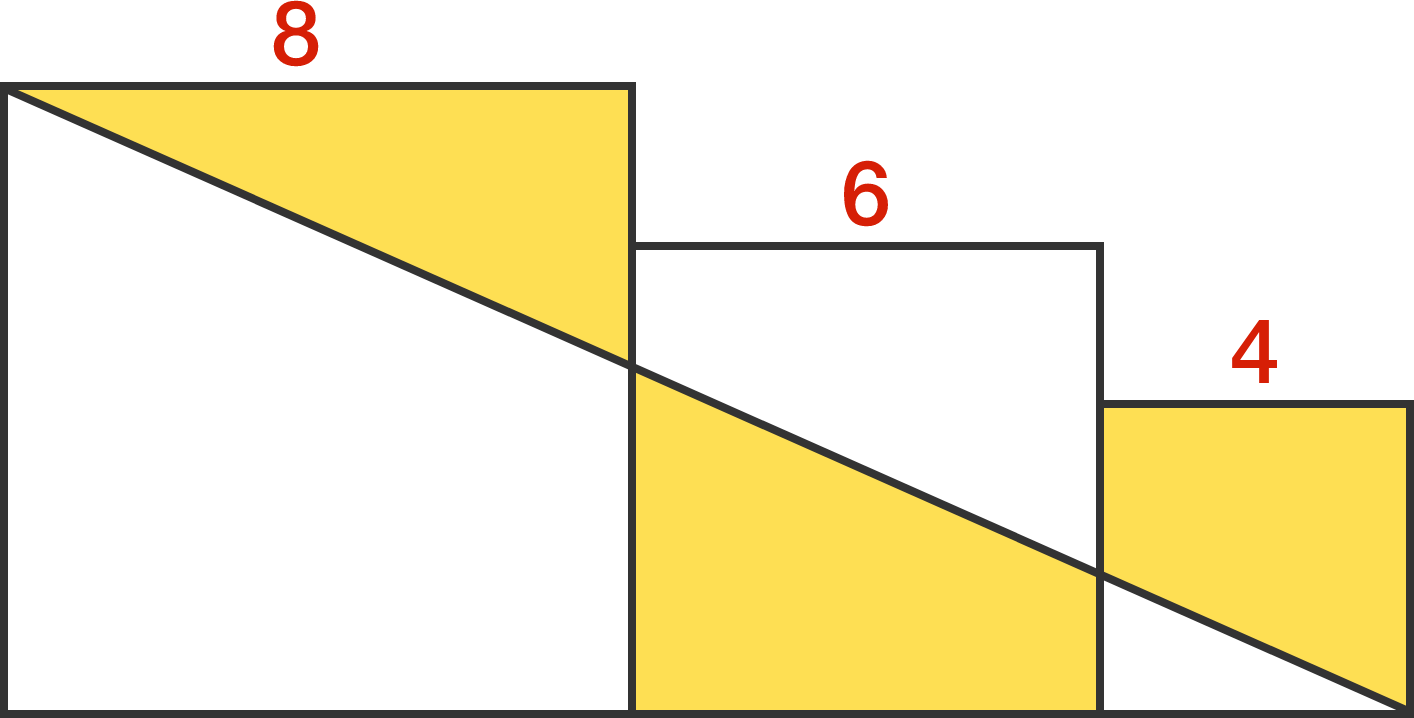# Finding the area

Geometry Level 3The side lengths of the squares in the diagram are $8, 6,$ and $4,$ respectively. Find the area of the yellow region.

If your answer can be expressed as $\frac{a}{b},$ where $a$ and $b$ are coprime positive integers, give your answer as $a+b.$

×

Problem Loading...

Note Loading...

Set Loading...